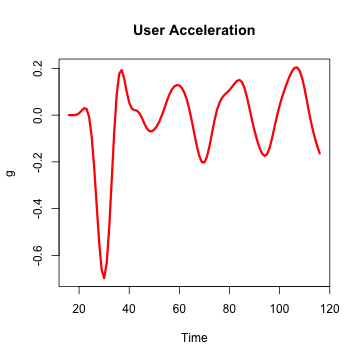# DSP for Data Analysis

## Brief introduction into digital signal processing

Mykola Pavlov
Founder at Biomech

## Topics

• What is DSP and where it can be applied?
• Linear systems
• Fourier analysis

## What is Signal?

Anything that carries information is a signal## What is DSP and where it can be applied?

The mathematics, the algorithms, and the techniques used to manipulate signals after they have been converted into a digital form.## Contineous and discrete

• Contineous f(t)
• Discrete (digital) f[t]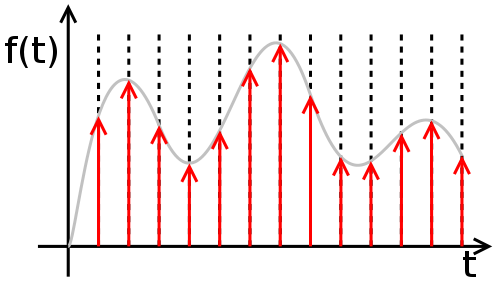## Linear system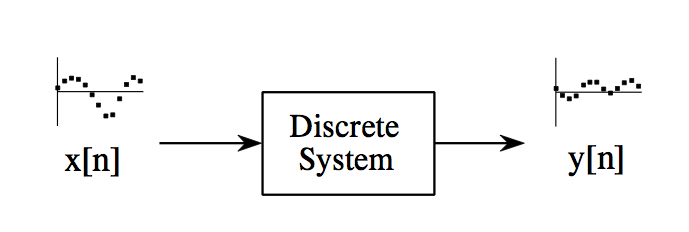Examples: Wave propagation, resistors, capacitors, inductors, amplifiers, filters, mechanical interaction of masses, springs, echoes, resonances, image blurring...

## Linear system properties: Homogenity

Amplitude change in the input results in an identical amplitude change in the output.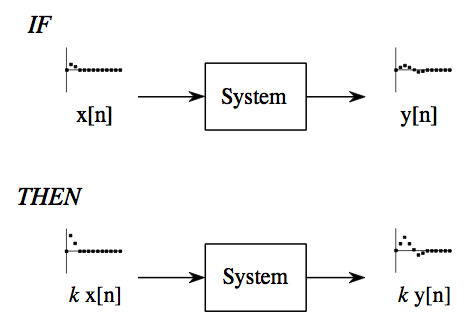Signals added at the input produce signals that are added at the output.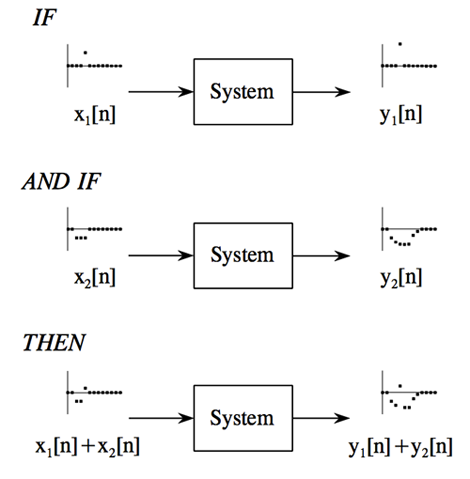## Linear system properties: Shift invariance

Shift in the input signal causes an identical shift in the output signal.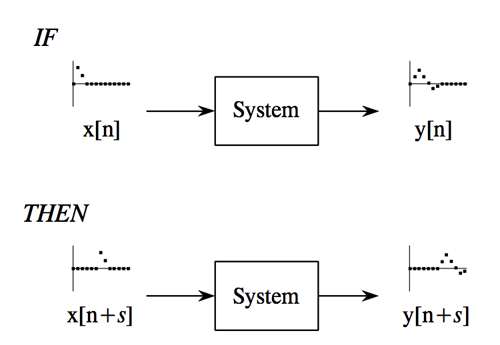## Superposition strategy: divide-and-conquer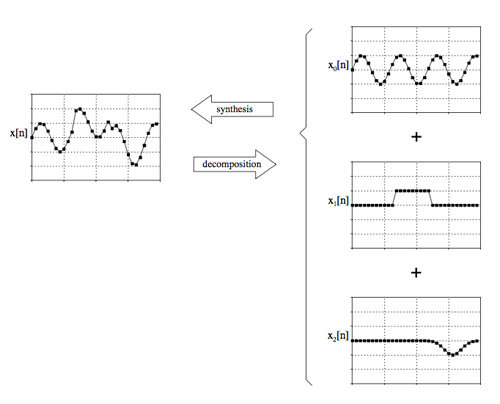``````Synthesis: 5 + 10 = 15
Decomposition: 15 = 3 + 12 or 1 + 5 + 9 or ...
``````

## Superposition strategy: divide-and-conquer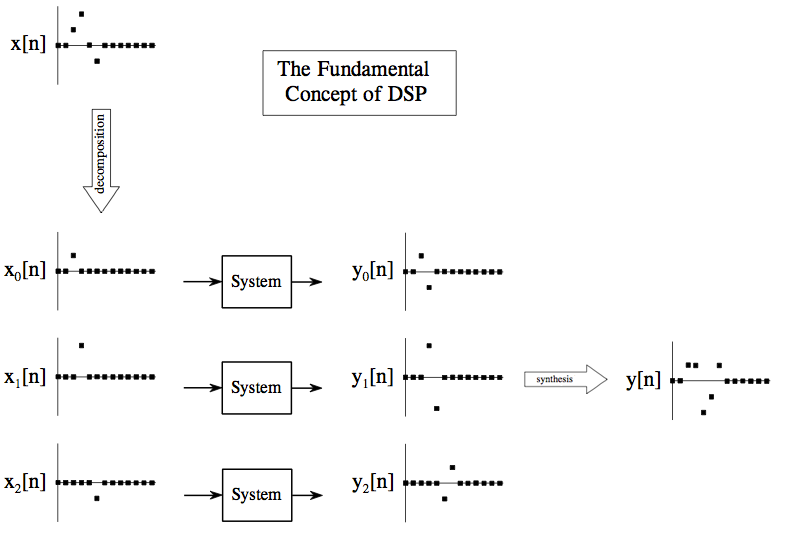## Superposition strategy: divide-and-conquer

``````Divide-and-conquer: 1027 * 4 = 1000 * 4 + 20 * 4 + 7 * 4
``````

## Two decompositions: Impulse and Fourier

### Impulse decomposition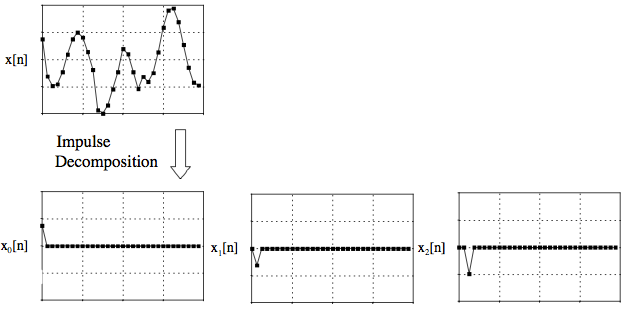## Delta function and Impulse Response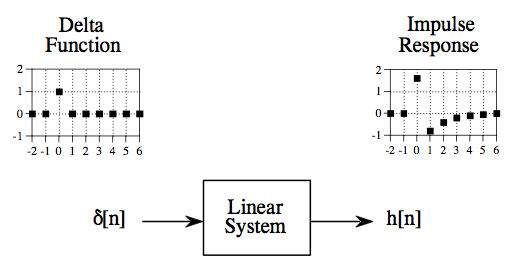Same as filter kernel, convolution kernel, kernel, point spread function (image processing).

## Let's summarize

1. Input signal can be decomposed into a set of impulses
2. Each impulse can be viewed as a scaled and shifted delta function
3. Output signal can be found by adding these scaled and shifted impulse responses (This operations is known as Convolution).

Intuition: If we know impulse response, then we can calculate the output for any possible input signal!

## Fourier decomposition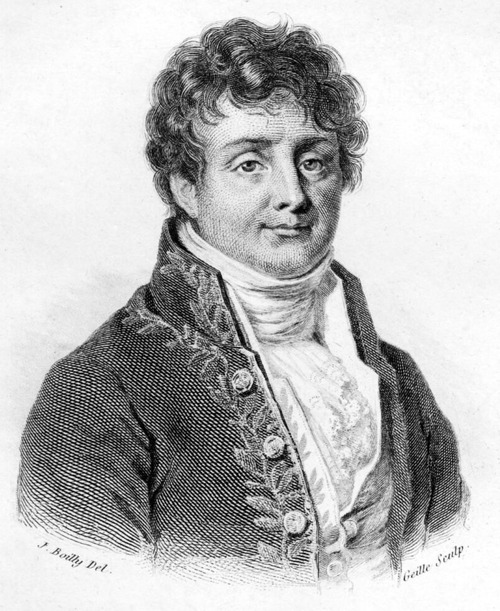## Fourier decomposition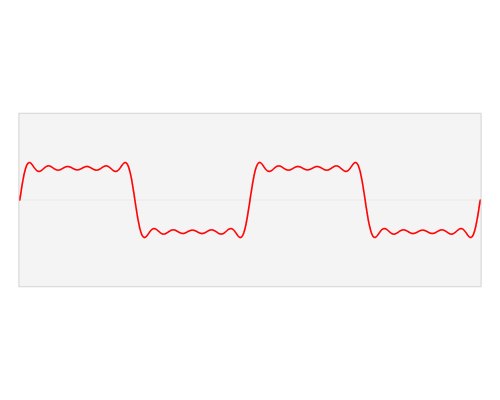## DFT, IDFT, FFT

``````signal <- rnorm(100)
fourier_transform <- fft(signal)

Re(fourier_transform)[1:5]
``````
``````##  -4.874 -3.616 -9.085  5.431 -2.516
``````
``````Im(fourier_transform)[1:5]
``````
``````##   0.0000 -0.9916 10.2477 -1.5758 -0.3789
``````
• Re[] - Cos, Index - Freq, Value - Amplitude
• Im[] - Sin, Index - Freq, Value - Amplitude

## Rectangular vs Polar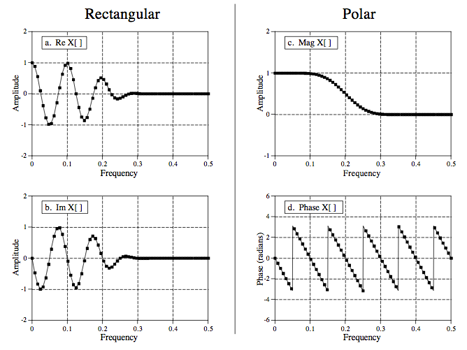``````Mod(fourier_transform)[1:5]
``````
``````##   4.874  3.749 13.695  5.655  2.545
``````

## Furier analysis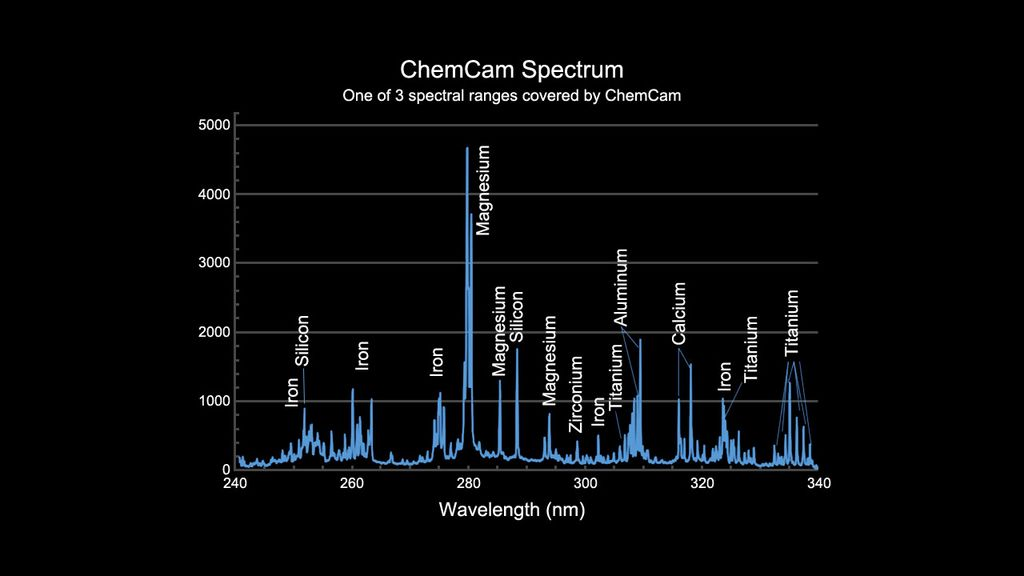## Filter design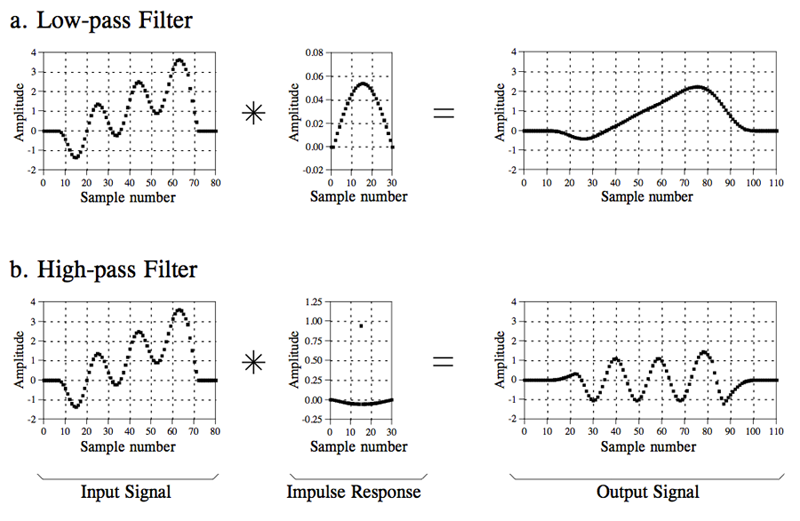## Filter types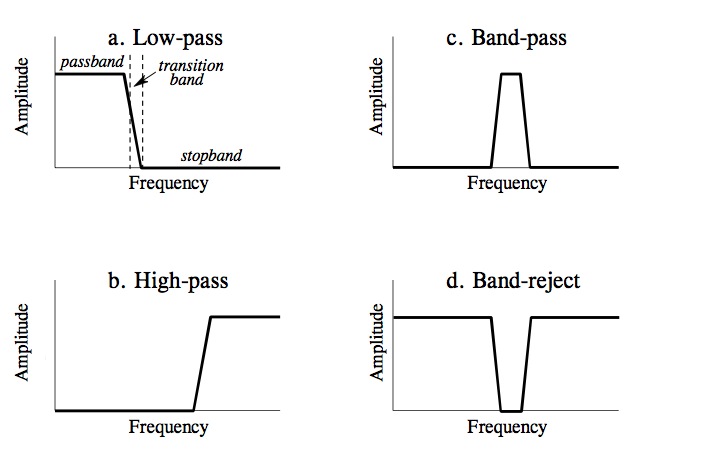## Filter types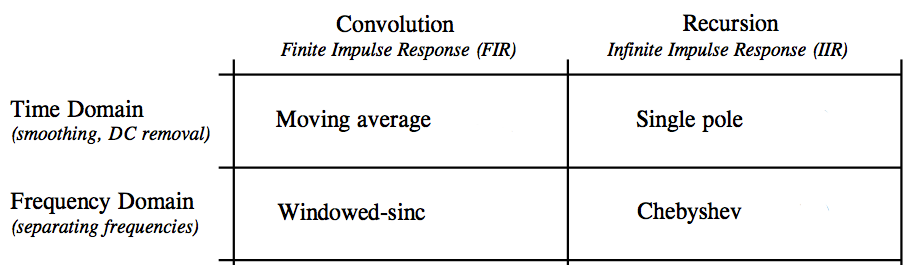## Window Sinc Filter

``````library(signal)
t <- as.vector(as.matrix(read.csv("data/data.csv", row.names = NULL, header = F)))  # 20 Hz
plot.ts(t, ylab = "g", main = "User Acceleration")
``````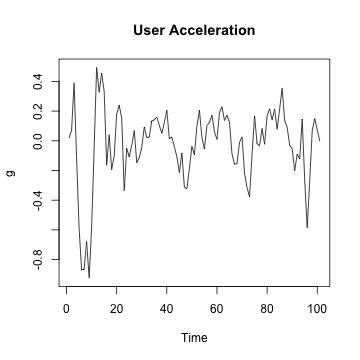## Window Sinc Filter

``````filt <- fir1(15, 0.15, type = "low")  # 3 Hz low-pass filter
z <- filter(filt, t)  # apply filter
plot(z, col = "red", lwd = 3, type = "l", ylab = "g", main = "User Acceleration")
``````